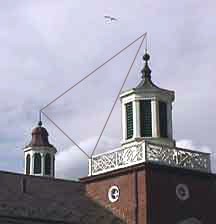See the picture.

# TrianglesA triangle is made by connecting three points in 3D space with line segments. Unless the three points all lie on the same line, three points in space define a unique triangle. Triangles in space are important in 3D graphics. The 3D objects that make up the scene are constructed out of triangles and other flat polygons.

The triangle in the 2D picture is a projection of the 3D triangle in the scene. The projection distorts the 3D triangle. The 3D triangle is much larger than the 2D triangle, and the angles are different.

Say that a 3D object in the scene is constructed of several triangles. Now project each triangle into a 2D picture. The result is the projected version of the 3D object. The white lattice work on the building is made up of many triangles. The image of the lattice in the picture shows all these triangles projected (by the lens of the camera).

These topics will be discussed in depth in your computer graphics book. Right now, just think about geometrical figures in space.

### QUESTION 7:

Do you need a coordinate frame (a system of labeling points with x, y, and z coordinates) to talk about points, lines, and plane figures?